# Very closeElectronics guide > Transistors > Very close

Unfortunately things aren’t quite as simple as that in electronic circuits, where individual resistances are rarely considered alone. The real life transistor deals with currents in more than one direction and this confuses the issue. However, all we need know here (thankfully) is that the two PN junctions are very close together. So close that, in fact, they affect one another. It’s almost as if they’re Siamese twins — and whatever happens to one affects the other.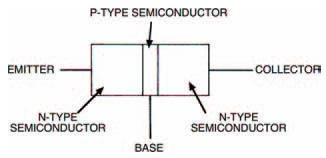Figure 8.3 A narrow P-area gives two PN junctions very close together

Figure 8.3 illustrates how a transistor can be built up, from two PN junctions situated very close together. It’s really only a thin layer of P-type semiconductor material (only a few hundred or so atoms thick) between two thicker layers of N-type semiconductor material. Now let’s connect this transistor arrangement between a voltage supply, so that collector is positive and emitter is zero, as in Figure 8.4.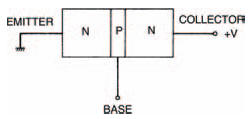Figure 8.4 Connected up, the emitter is at 0V and the collector is positive

From what we know so far, nothing can happen and no current can flow from collector to emitter because between these two terminals two back-to-back PN junctions lie. One of these junctions is reverse biased and so, like a reverse biased diode, cannot conduct. So, what’s the point of it all?

Well, as mentioned earlier, what happens in one of the two PN junctions of the transistor affects the other. Let’s say for example that we start the lower PN junction (between base and emitter) conducting by raising the base voltage so that the base-to-emitter voltage is above the transition voltage of the junction (say, 0V6 if the transistor is a silicon variety). Figure 8.5 shows this situation. Now, the lower junction is flooded with charge carriers and because both junctions are very close together, these charge carriers allow current flow from collector to emitter also, as shown in Figure 8.6.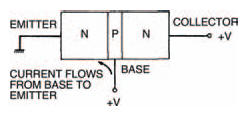Figure 8.5 If the voltage at the base is raised, current flows from base to emitterFigure 8.6 Charge carriers accumulating around the lower junction allow current to flow from the collector to the emitter

So, to summarise, a current will flow from collector to emitter of the transistor when the lower junction is forward biased by a small base-to-emitter voltage. When the base-to-emitter voltage is removed the collector-toemitter current will stop.

We can build a circuit to see if this is true, as shown in Figure 8.7. Note the transistor circuit symbol. Figure 8.8 shows the breadboard layout. From the circuit you’ll see that we’re measuring the transistor’s collector-toemitter current (commonly shortened to just collector current) when the base-to-emitter junction is first connected to zero volts and to all intents and purposes is reverse biased, then when the base is connected to positive and the baseemitter junction is forward biased.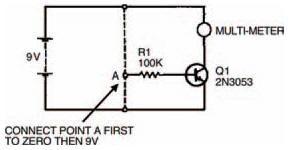Figure 8.7 An experimental circuit to test what we have described so far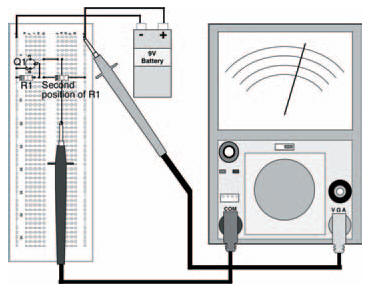Figure 8.8 A breadboard layout for the circuit in Figure 8.7

Now do the experiment and see what happens. You should find that when the base is connected to zero via the 100 kΩ resistor nothing is measured by the multimeter. But when the base resistor is connected to positive, the multi-meter shows a collector current flow. In our experiment a collector current of about 12 mA was measured — yours may be a little different. Finally, when the base resistor is returned to zero volts (or simply when it’s disconnected!) the collector current again does not flow.

So what? What use is this? Not a lot as it stands, but it becomes very important when we calculate the currents involved. We already know the collector current (about 12 mA in our case) but what about the base-to-emitter current (shortened to base current)? The best way to find this is not by measurement (the meter itself would affect the transistor’s operation!) but by calculation. We know the transistor’s base-to-emitter voltage (shortened to base voltage) and we know the supply voltage.

From these we can calculate the voltage across the resistor, and from Ohm’s law we can therefore calculate the current through the resistor. And the current through the resistor must be the base current.

The resistor voltage is: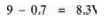So, from Ohm’s law the current is: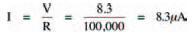Not a lot!

Now we can begin to see the importance of the transistor. A tiny base current can turn on or off a quite large collector current. This is illustrated in Figure 8.9 and is of vital importance — so remember it!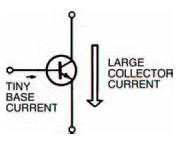Figure 8.9 Important: a very small base current can control a large collector current

In effect, the transistor is a current amplifier. No matter how small the base current is, the collector current will be much larger. The collector current is, in fact, directly proportional to the base current. Double the base current and you double the collector current. Halve the base current and the collector current is likewise halved. It’s this fact that the transistor may be used as a controlling element (the base current controlling the collector current) which makes it the most important component in the electronics world.

The ratio: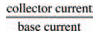gives a constant of proportionality for the transistor, which can have many names depending on which way you butter your bread. Officially it’s called the forward current transfer ratio, common emitter, but as that’s quite a mouthful it’s often just called the transistor’s current gain (seems sensible!). You can sometimes shorten this even further if you wish, to the symbols: hfe, or ß. In manufacturer’s data sheets for transistors the current gain is normally just given the symbol hfe (which if you’re interested stands for Hybrid parameter, Forward, common Emitter. Are you any wiser?). However, we’ll just stick to the term current gain, generally.

We can work out the current gain of a transistor by measuring the collector current, and calculating the base current as we did earlier, and dividing one by the other. For example the current gain of the transistor we used is:Yours may be a bit different. Manufacturers will quote typical values of current gain in their data sheets — individual transistors’ current gains will be somewhere around this value, and may not be exact at all. It really doesn’t matter, too much. The transistor we use here, the 2N3053, is a fairly common general-purpose transistor. High power transistors may have current gains more in the region of about 10, while some modern transistors for use in high frequency circuits such as radio may have current gains around 1000 or so.

<< Transistors